AP State Syllabus AP Board 6th Class Maths Solutions Chapter 5 Fractions and Decimals InText Questions and Answers.

## AP State Syllabus 6th Class Maths Solutions 5th Lesson Fractions and Decimals InText Questions(Page No. 63)

Question 1.
Is it true to say that 3 × $$\frac{1}{5}=\frac{1}{5}$$ x 3?
Solution:
3 × $$\frac{1}{5}=\frac{1}{5}$$ × 3. Yes, it is true.
By using commutative property over multiplication a × b = b × a
3 × $$\frac{1}{5}=\frac{1}{5}$$ × 3 = $$\frac{3}{5}$$

Check Your Progress (Page No. 63)

Find :
i) $$5 \times 3 \frac{2}{7}$$
ii) $$2 \frac{5}{9} \times 3$$
iii) $$2 \frac{4}{7} \times 3$$
iv) $$3 \times 1 \frac{3}{4}$$
Solution: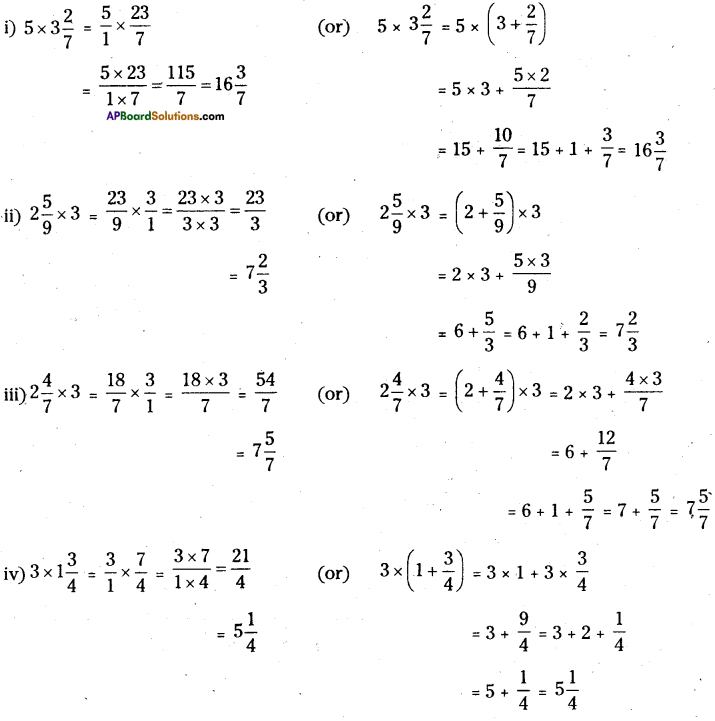Let’s Explore (Page No. 64)

Question 1.
Observe the products of fractions.
Have you observed the products of any two fractions is always lesser or greater than each of its fraction, write conclusion.
$$\frac{1}{5} \times \frac{2}{3}=\frac{2}{15}$$ (Product of two proper fractions)
Solution:
Product of any two proper fractions is always less than each of its fraction.
i. e., $$\frac{2}{15}<\frac{1}{5} \text { and } \frac{2}{15}<\frac{2}{3}$$

ii) $$\frac{3}{2} \times \frac{5}{4}=\frac{15}{8}$$ (Product of two improper fractions) •
Solution:
The product of any two improper fractions is always greater than each of its fraction.
i.e, $$\frac{3}{2}<\frac{15}{8} \text { and } \frac{5}{4}<\frac{15}{8}$$

iii) $$\frac{2}{3} \times \frac{5}{3}=\frac{10}{9}$$ (Product of proper and improper fractions)
Solution:
The product of a proper fraction and an improper fraction is always greater than its proper fraction and less than its improper fraction.
i.e., $$\frac{2}{3}<\frac{10}{9} \text { and } \frac{5}{3}>\frac{10}{9}$$(Pg. No. 66)

Question 1.
i) 4 ÷ $$\frac{1}{8}$$
ii) 9 ÷ $$\frac{3}{4}$$
iii) 7 ÷ $$\frac{2}{3}$$
iv) 35 ÷ $$\frac{7}{3}$$
v) 4 ÷ $$\frac{15}{8}$$
Solution: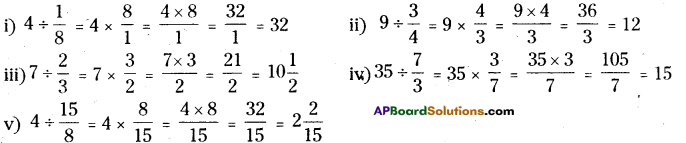(Pg. No. 67)

Question 1.
Observation these products and fill in the blanks.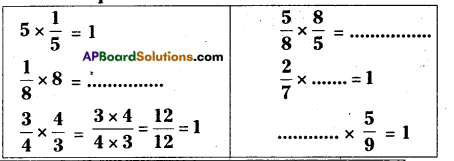Solution: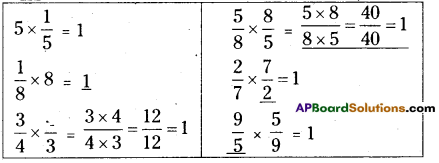Check Your Progress (Page No. 68)

Question 1.
Write the reciprocal of fractions in the given table.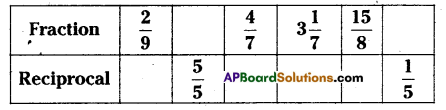Solution: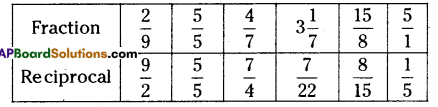(Reciprocal of a fraction $$\frac{\mathrm{a}}{\mathrm{b}}$$ is $$\frac{\mathrm{b}}{\mathrm{a}}$$)(Page No. 69)

Question 1.
Find
i) $$\frac{7}{9}$$ ÷ 4
ii) $$\frac{3}{4}$$ ÷ 9
iii) 4$$\frac{1}{2}$$ ÷ 6
iv) $$\frac{1}{5}$$ ÷ 3
Solution: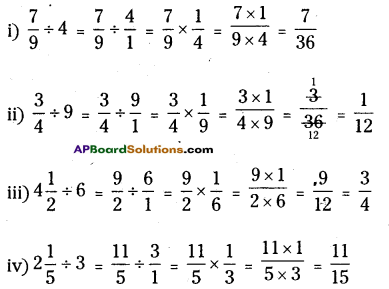Check Your Progress (Page No. 73, 74 & 75)

Question 1.
Fill in the blanks.

 Fraction Decimal Number ” Read as $$\frac{6}{10}$$ 0.6 Zero point six $$\frac{37}{100}$$ 0.37 Zero point three seven 0.721 Zero point seven two one Seventeen point two

Solution:

 Fraction Decimal Number Read as $$\frac{6}{10}$$ 0.6 Zero point six $$\frac{37}{100}$$ 0.37 Zero point three seven $$\frac{721}{1000}$$ 0.721 Zero point seven two one $$\frac{172}{10}$$ 17.2 Seventeen point two

Question 2.
Write the place value of the circled digits.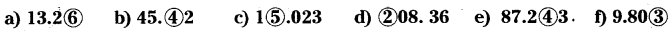Solution: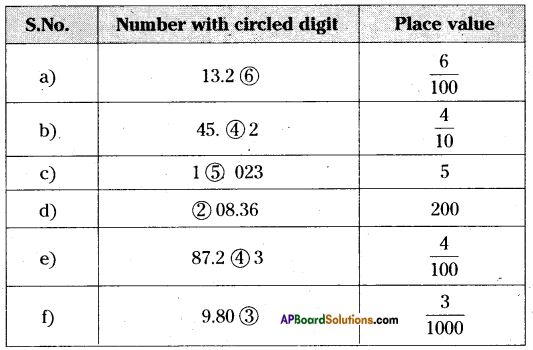Question 3.
a) 700 + 40 + 2 + $$\frac{1}{10}+\frac{3}{100}+\frac{6}{1000}$$
Solution:
700 + 40 + 2 + 0.1 + 0.03 + 0.006 = 742.136

b) 9000 + 800 + 3 + 0.2 + 0.05 + 0.007
Solution:
9000 + 800 + 3 + 0.2 + 0.05 + 0.007 = 983.257

c) 6000 + 400 + 20 + 1 + $$\frac{2}{10}+\frac{5}{100}+\frac{9}{1000}$$
Solution:
6000 + 400 + 20 + 1 + 0.2 + 0.05 + 0.009 = 6421. 259

d) 400 + 5+ $$\frac{1}{10}+\frac{8}{100}$$
Solution:
400 + 5 + 0.1 +0.08 = 405.18Question 4.
Expand the following into decimals and fractional forms,
a) 164.238
b) 968.054
Solution:
a) 164.238 = 100 + 60 + 4 + 0.2 + 0.03 + 0.008
= 100 + 60 + 4 + $$\frac{2}{10}+\frac{3}{100}+\frac{8}{1000}$$

b) 968.054
Solution:
= 900 + 60 + 8 + 0.0 + 0.05 + 0.004
= 900 + 60 + 8 + 0 + $$\frac{5}{100}+\frac{4}{1000}$$
= 900 + 60 + 8 + $$\frac{5}{100}+\frac{4}{1000}$$

Question 5.
Write fractions as decimals.
1. $$\frac{23}{10}$$ = ………..
2. $$\frac{6}{100}$$ = ………..
3. $$\frac{3}{8}$$ = ………..
4. $$\frac{2}{25}$$ = ………..
Solution:
1. $$\frac{23}{10}$$ = 2.3
2. $$\frac{6}{100}$$ = 0.06
3. $$\frac{3}{8}$$ = 0.375
4. $$\frac{2}{25}$$ = 0.08Question 6.
Write decimals as fractions in simplest form.
1. 0.2 = ……………
2. 0.38 = ……………
3. 1.62 = ……………
4. 8.1 = ……………
Solution:
1. 0.2 = $$\frac{2}{10}$$
2. 0.38 = $$\frac{38}{100}$$
3. 1.62 = $$\frac{162}{100}$$
4. 8.1 = $$\frac{81}{10}$$